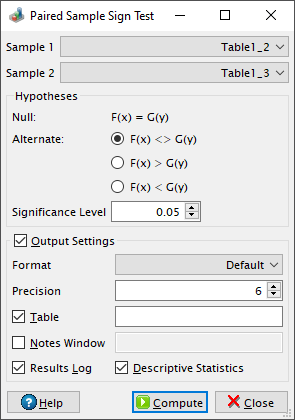# Sign Test Dialog

This dialog is activated by selecting the Paired Sample Sign Test... command from the Statistics -> Nonparametric Tests -> menu. It can be used in order to test for consistent differences between pairs of observations. The Wikipedia article on the sign test makes for excellent reading on this topic.

Figure 5-108. The paired sample sign test dialog.For two paired samples, `Xi` and `Yi`, the test statistics (`S`) is the number of pairs for which Xi < Yi. QtiPlot also computes the reduced sample size (`Nr`), which is the number of non-tied paires (Xi ≠ Yi) and a probability `p`.

For a lower tail test, the p-value is the probability of observing a value ≤ S, if S ≤ Nr/2, or of observing a value < S, if S > Nr/2 and is calculated using the cumulative distribution function of the binomial distribution: binocdf(S, Nr, 0.5). For an upper tailed test, the probability is calculated as the complement of the lower tail probability: 1-p. For a two-tailed test, the probability is calculated as the double of the lower of these two values: 2min(p, 1-p). The null hypothesis is rejected if the probability is lower than the value of the significance level.

If Nr > 25, QtiPlot also computes a Z-score, using the following formula: Z = [S-m-0.5sign(S-m)]/Var1/2, where 0.5 is a continuity correction factor, m = Nrp is the mean of the binomial distribution with probability p and its variance is Var = Nrp(1-p). In our case positive and negative differences are equally probable for the paired samples, therefore p is 0.5.

If there are missing values in the two source data columns, the whole pair will be excluded from the analysis.